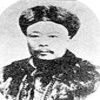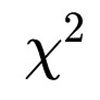#### You may also like### Very Old Man

Is the age of this very old man statistically believable?### Reaction Timer Timer

How can you time the reaction timer?### Chi-squared Faker

How would you massage the data in this Chi-squared test to both accept and reject the hypothesis?

# Robin's Hypothesis Testing

##### Age 16 to 18Challenge Level
Robin has a bag containing red and green balls.  Robin wants to test the following hypotheses, where $\pi$ is the proportion of green balls in the bag:

$H_0\colon \pi=\frac{1}{2}$  and  $H_1\colon \pi\ne\frac{1}{2}$

Robin is allowed to take out a ball at random, note its colour and then replace it: this is called a trial.  Robin can do lots of trials, but each trial has a certain cost.

Robin wants to test these hypotheses as cheaply as possible, so suggests the following approach:

"I will do at most 50 trials.  If the p-value* drops below 0.05 at any point, then I will stop and reject the null hypothesis at the 5% significance level, otherwise I will accept it."

Warning - the computer needs a little bit of thinking time to do the simulations!

In this simulation, you can:
• specify the number of green and red balls actually in the bag (and the true ratio is shown with a green dashed line on the graph) - note that in a real experiment we would not know this!
• specify the number of trials (up to 200)
• specify the proportion for the null hypothesis (which we took to be $\frac{1}{2}$ above)
• choose whether to show the proportion of green balls after each ball is picked
• choose whether to show the p-value after each ball is picked*
• rerun the simulation ("Repeat experiment")
The "Final p-value" shows the p-value at the end of the experiment, and the orange lines are at 0.1, 0.05 and 0.01.

Here are some questions you could consider as you think about Robin's approach:
• What do you notice about the patterns of proportions and p-values?  Is there anything which is the same every time or most times you run the simulation?
• If we repeat the experiment lots of times, how often does $H_0$ get rejected using Robin's approach?  Does the answer to this depend on how many trials we perform?
• Does the answer change if you change the true proportion of greens in the bag?
• What would happen if you changed the hypothesised proportion $\pi$?
• What would happen if you changed the significance level from 5% to 10% or 1%?
You may want to ask and explore other questions as well.

Rejecting $H_0$ when it is true is called a Type I error.

* To read more about p-values, have a look at What is a Hypothesis Test?  The p-values here are calculated like this: after $k$ trials, we find twice the probability of obtaining this number of greens or a more extreme number in $k$ trials, assuming that $H_0$ is true.  The graph shows how this p-value changes with $k$.

This resource was inspired by the controversy surrounding a paper published in Nature Communications, as discussed by Casper Albers here.

This resource is part of the collection Statistics - Maths of Real Life